# Algebra - math word problems

#### Number of problems found: 2648

• Hexagonal pyramidPlease calculate the height of a regular hexagonal pyramid with a base edge of 5cm and a wall height of w = 20cm. Please sketch a picture.
• Mary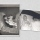Mary is 12 years old, which is 40% of the age of her mother. How many percent of her mother's age will Mary have after the next 12 years?
• Trio ratioHans, Alena and Thomas have a total of 740 USD. Hans and Alena split in the ratio 5: 6 and Alena and Thomas in the ratio 4: 5. How much will everyone get?
• Christmas or EasterPlease calculate this example by the Venn equation. They asked 73 students whether they like Christmas or Easter. 34 of them like one of the holidays. 39 loves Easter. There are twice as many students who wish both holidays than those who only love Ea
• The aspect ratioThe aspect ratio of the rectangular triangle is 13: 12: 5. Calculate the internal angles of the triangle.
• Twenty-fiveHow many three-digit natural numbers are divisible by 25?
• The schoolyardThe schoolyard had the shape of a square with an 11m side. The yard has been enlarged by 75 m2 and has a square shape again. How many meters was each side of the yard enlarged?
• Eq triangle minus arcsIn an equilateral triangle with a 2cm side, the arcs of three circles are drawn from the centers at the vertices and radii 1cm. Calculate the content of the shaded part - a formation that makes up the difference between the triangle area and circular cuts
• Pilsen circus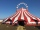The arrival of the circus in Pilsen was seen in the morning at 08:00 by a citizen of the city. He passed this information on 08:15 to three other residents of the city. Each of these three people then informed the other three residents at 08:30, and again
• Intelligence testPaľo, Jano, Karol, and Rišo were doing an intelligence test. Palo correctly answered half of the questions plus 7 questions, Jano to a third plus 18 questions, Karol to a quarter plus 21 questions and Risho to a fifth plus 25 questions. After the test, K
• The farm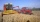The farm harvested 390 tons of grain. Wheat was 15% more than barley, and rye was 126 tons less than wheat and barley combined. How many tons of wheat, how many tons of barley, and how much rye did the team get?
• Pilsen RegionBetween 2000 and 2001, 14 per mille of the population decreased in the Pilsen Region. In 2000, the Pilsen Region had 551281 inhabitants. If the declining trend continues the same (i. E. , 14 per mille of inhabitants per year), how many inhabitants will th
• Two patches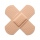Peter taped the wound with two rectangular patches (one over the other to form the letter X). The area sealed with both patches at the same time had a content of 40cm2 and a circumference of 30cm. One of the patches was 8cm wide. What was the width of the
• Two-digit numberDigit sum of thinking two-digit natural number is 11. When it exchanging a sequence of digits, given a number which is 27 less than the thinking number. Find out which number I think.
• Tributaries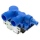The pool can be filled with two different tributaries. The first inflow would fill the pool in 18 hours, both in 6 hours. How many hours would the pool filled with a second inflow?
• The perimeter of the rectangleThe length, l, of a rectangle is 4 inches greater than its width, w. The perimeter of the rectangle is at least 30 inches. What inequality shows the range of possible widths of the rectangle?
• QuotientFind quotient before the bracket - the largest divisor 51 a + 34 b + 68 121y-99z-33
• Two machinesThe first machine can produce parts in 24 hours, the second in 16 hours. The first started working in the morning at 6:00 pm. Second at 10:00 o'clock. At what hour were all parts made?
• Pouring alcohol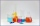100 liters of alcohol has 70% How many liters of water need to be added to have 60% alcohol?
• Two numbersThe difference between the two numbers is 74. If we divide a larger number by a smaller one, we get a quotient 7 and the rest of 2. Determine both numbers.

Do you have an interesting mathematical word problem that you can't solve it? Submit a math problem, and we can try to solve it.

We will send a solution to your e-mail address. Solved examples are also published here. Please enter the e-mail correctly and check whether you don't have a full mailbox.

Please do not submit problems from current active competitions such as Mathematical Olympiad, correspondence seminars etc...